Test: Energy Equation & Its Applications - 2

# Test: Energy Equation & Its Applications - 2 - Civil Engineering (CE)

Test Description

## 9 Questions MCQ Test GATE Civil Engineering (CE) 2024 Mock Test Series - Test: Energy Equation & Its Applications - 2

Test: Energy Equation & Its Applications - 2 for Civil Engineering (CE) 2023 is part of GATE Civil Engineering (CE) 2024 Mock Test Series preparation. The Test: Energy Equation & Its Applications - 2 questions and answers have been prepared according to the Civil Engineering (CE) exam syllabus.The Test: Energy Equation & Its Applications - 2 MCQs are made for Civil Engineering (CE) 2023 Exam. Find important definitions, questions, notes, meanings, examples, exercises, MCQs and online tests for Test: Energy Equation & Its Applications - 2 below.
Solutions of Test: Energy Equation & Its Applications - 2 questions in English are available as part of our GATE Civil Engineering (CE) 2024 Mock Test Series for Civil Engineering (CE) & Test: Energy Equation & Its Applications - 2 solutions in Hindi for GATE Civil Engineering (CE) 2024 Mock Test Series course. Download more important topics, notes, lectures and mock test series for Civil Engineering (CE) Exam by signing up for free. Attempt Test: Energy Equation & Its Applications - 2 | 9 questions in 30 minutes | Mock test for Civil Engineering (CE) preparation | Free important questions MCQ to study GATE Civil Engineering (CE) 2024 Mock Test Series for Civil Engineering (CE) Exam | Download free PDF with solutions
 1 Crore+ students have signed up on EduRev. Have you?
Test: Energy Equation & Its Applications - 2 - Question 1

### If a1 and a2 are the cross-sectional areas of a tank and orifice, h the height of water level intank above the centre of the orifice, the velocity of approach is given by

Test: Energy Equation & Its Applications - 2 - Question 2

### If the coefficient of velocity of flow through a orifice is cv and coefficient of resistance is cr, then

Detailed Solution for Test: Energy Equation & Its Applications - 2 - Question 2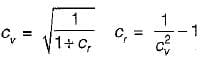Test: Energy Equation & Its Applications - 2 - Question 3

### If Cv, Cc, Dd and Cr are the hydraulic coefficients of an orifice, then

Test: Energy Equation & Its Applications - 2 - Question 4

The momentum correction factor for triangular velocity distribution for flow between parallel plates as shown in the figure is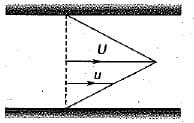Detailed Solution for Test: Energy Equation & Its Applications - 2 - Question 4

Triangular velocity distribution is achieved for Couette flow.
Average velocity,Vav = V/2
Momentum correction factor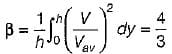Energy correction factor,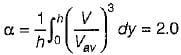Test: Energy Equation & Its Applications - 2 - Question 5

If the velocity is zero over half of the cross- sectionai area and is uniform over the remaining half, then the momentum correction factor is

Detailed Solution for Test: Energy Equation & Its Applications - 2 - Question 5

Momentum correction factor,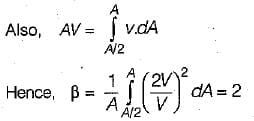Test: Energy Equation & Its Applications - 2 - Question 6

Match List-I with List-I and select the correct answer using the codes given below the lists:
List-I
A. Moment of momentum equation
B. Bernoulli’s equation
C. Euler’s equation
D. Hagen-Poiseuille equation

List-II
1. Equation to find energy loss in a pipeline
2. Equation of motion for one-dimensional steady flow of ideal and incompressible fluid
3. Equation based on conservation of momentum principle applicable to circulatory flows
4. Three-dimensional equation ofmotion based on Principle of conservation of momentum for ideal and incompressible fluid flow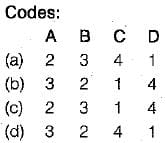Detailed Solution for Test: Energy Equation & Its Applications - 2 - Question 6

Bernoulli's equation is derived from equation of motion for one-dimensional steady flow of ideal and incompressible fluid. \

Test: Energy Equation & Its Applications - 2 - Question 7

If velocity is zero over 1/3rd of a cross-section and is uniform over remaining 2/3rd of the cross-section, then the correction factor for kinetic energy is

Detailed Solution for Test: Energy Equation & Its Applications - 2 - Question 7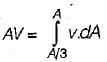∴ v = 3/2 v
∴ Kinetic energy correction factor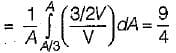Test: Energy Equation & Its Applications - 2 - Question 8

A jet of water issue from a 5cm diameter nozzle, held vertically upwards, at a velocity of 20 m/sec. If air resistance consumes 10% of the initial energy of the jet, then it would reach a height, above the nozzle, of

Detailed Solution for Test: Energy Equation & Its Applications - 2 - Question 8

Using Bernoulli’s equation,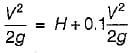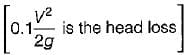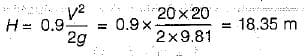Test: Energy Equation & Its Applications - 2 - Question 9

The kinetic energy correction factor is

## GATE Civil Engineering (CE) 2024 Mock Test Series

28 docs|296 tests
Information about Test: Energy Equation & Its Applications - 2 Page
In this test you can find the Exam questions for Test: Energy Equation & Its Applications - 2 solved & explained in the simplest way possible. Besides giving Questions and answers for Test: Energy Equation & Its Applications - 2, EduRev gives you an ample number of Online tests for practice

## GATE Civil Engineering (CE) 2024 Mock Test Series

28 docs|296 tests